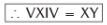Courses

# Olympiad Test: Roman Numerals -2

## 10 Questions MCQ Test Mathematics Olympiad Class 4 | Olympiad Test: Roman Numerals -2

Description
This mock test of Olympiad Test: Roman Numerals -2 for Class 4 helps you for every Class 4 entrance exam. This contains 10 Multiple Choice Questions for Class 4 Olympiad Test: Roman Numerals -2 (mcq) to study with solutions a complete question bank. The solved questions answers in this Olympiad Test: Roman Numerals -2 quiz give you a good mix of easy questions and tough questions. Class 4 students definitely take this Olympiad Test: Roman Numerals -2 exercise for a better result in the exam. You can find other Olympiad Test: Roman Numerals -2 extra questions, long questions & short questions for Class 4 on EduRev as well by searching above.
QUESTION: 1

Solution:
QUESTION: 2

Solution:
QUESTION: 3

### Write 630 as a roman numeral.

Solution:
QUESTION: 4

Write 830 as a roman numeral.

Solution:

We have
DCCCXXX = 500 + 100 + 100 + 100
+ 10 + 10 + 10 = 830

QUESTION: 5

Write 737 as a roman numeral.

Solution:
QUESTION: 6

Write 2676 as a roman numeral.

Solution:
QUESTION: 7

Write 2990 as a roman numeral.

Solution:
QUESTION: 8

Convert the statement into Roman numbers 5 × 4.

Solution:

Here 5 × 4 = VXIV
and 5 × 4 = 20 = XXQUESTION: 9

Solve XII – II

Solution:

We have XII – II = 12 – 2 = 10

QUESTION: 10

Solve DVI – XXIV = ?

Solution:

We have DVI – XXIV = 500 + 6 – 14
= 506 – 24
and CDL XXX II
= (500 – 100) + 50 + 10 + 10 + 10 + 2
= 482